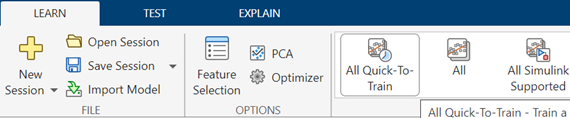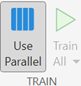## Train Regression Models in Regression Learner App

You can use Regression Learner to train regression models including linear regression models, regression trees, Gaussian process regression models, support vector machines, ensembles of regression trees, and neural network regression models. In addition to training models, you can explore your data, select features, specify validation schemes, and evaluate results. You can export a model to the workspace to use the model with new data or generate MATLAB® code to learn about programmatic regression.

Training a model in Regression Learner consists of two parts:

• Validated Model: Train a model with a validation scheme. By default, the app protects against overfitting by applying cross-validation. Alternatively, you can choose holdout validation. The validated model is visible in the app.

• Full Model: Train a model on full data without validation. The app trains this model simultaneously with the validated model. However, the model trained on full data is not visible in the app. When you choose a regression model to export to the workspace, Regression Learner exports the full model.

The app displays the results of the validated model. Diagnostic measures, such as model accuracy, and plots, such as a response plot or residuals plot, reflect the validated model results. You can automatically train one or more regression models, compare validation results, and choose the best model that works for your regression problem. When you choose a model to export to the workspace, Regression Learner exports the full model. Because Regression Learner creates a model object of the full model during training, you experience no lag time when you export the model. You can use the exported model to make predictions on new data.

To get started by training a selection of model types, see Automated Regression Model Training. If you already know which regression model you want to train, see Manual Regression Model Training.

### Automated Regression Model Training

You can use Regression Learner to automatically train a selection of different regression models on your data.

• Get started by automatically training multiple models simultaneously. You can quickly try a selection of models, and then explore promising models interactively.

• If you already know what model type you want, then you can train individual models instead. See Manual Regression Model Training.

1. On the Apps tab, in the Machine Learning and Deep Learning group, click Regression Learner.

2. Click and select data from the workspace or from a file. Specify a response variable and variables to use as predictors. See Select Data and Validation for Regression Problem.

3. On the Regression Learner tab, in the Model Type section, click the arrow to expand the list of regression models. Select All Quick-To-Train. This option trains all the model presets that are fast to fit.4. Click .

Note

If you have Parallel Computing Toolbox™, you can train the models in parallel. See Parallel Regression Model Training.

A selection of model types appears in the Models pane. When the models finish training, the best RMSE (Validation) score is highlighted in a box.5. Click models in the Models pane and open the corresponding plots to explore the results.

For the next steps, see Manual Regression Model Training or Compare and Improve Regression Models.

6. To try all the nonoptimizable model presets available, click, and then click Train.### Manual Regression Model Training

To explore individual model types, you can train models one at a time or train a group of models of the same type.

1. Choose a model type. On the Regression Learner tab, in the Model Type section, click a model type. To see all available model options, click the arrow in the Model Type section to expand the list of regression models. The nonoptimizable model options in the gallery are preset starting points with different settings, suitable for a range of different regression problems.

To read descriptions of the models, switch to the details view or place the mouse over a button to display its tooltip.2. After selecting a model, click .Repeat to explore different models.

Tip

Select regression trees first. If your trained models do not predict the response accurately enough, then try other models with higher flexibility. To avoid overfitting, look for a less flexible model that provides sufficient accuracy.

3. If you want to try all nonoptimizable models of the same or different types, then select one of the All options in the gallery.

Alternatively, if you want to automatically tune hyperparameters of a specific model type, select the corresponding Optimizable model and perform hyperparameter optimization. For more information, see Hyperparameter Optimization in Regression Learner App.

For next steps, see Compare and Improve Regression Models.

### Parallel Regression Model Training

You can train models in parallel using Regression Learner if you have Parallel Computing Toolbox. Parallel training allows you to train multiple models simultaneously and continue working.

To control parallel training, toggle the button on the app toolstrip. The button is available only if you have Parallel Computing Toolbox.The first time you click after clicking the button, a dialog box is displayed while the app opens a parallel pool of workers. After the pool opens, you can train multiple models at once.

When models are training in parallel, progress indicators appear on each training and queued model in the Models pane. If you want, you can cancel individual models. During training, you can examine results and plots from models, and initiate training of more models.

If you have Parallel Computing Toolbox, then parallel training is available in Regression Learner, and you do not need to set the `UseParallel` option of the `statset` function.

Note

You cannot perform hyperparameter optimization in parallel. The app disables the button when you select an optimizable model. If you then select a nonoptimizable model, the button is off by default.

### Compare and Improve Regression Models

1. Examine the RMSE (Validation) score reported in the Models pane for each model. Click models in the Models pane and open the corresponding plots to explore the results. Compare model performance by inspecting results in the plots. You can rearrange the layout of the plots to compare results across multiple models: use the options in the button, drag and drop plots, or select the options provided by the Document Actions arrowlocated to the right of the model plot tabs.

Additionally, you can compare the models by using the Sort by options in the Models pane. Delete any unwanted model by selecting the model and clicking the button in the upper right of the pane, or right-clicking the model and selecting Delete model.

2. Select the best model in the Models pane and then try including and excluding different features in the model. Click Feature Selection.Try the response plot to help you identify features to remove. See if you can improve the model by removing features with low predictive power. Specify predictors to include in the model, and train new models using the new options. Compare results among the models in the Models pane.

You also can try transforming features with PCA to reduce dimensionality.3. Improve the model further by changing model parameter settings in the Advanced dialog box. Then, train using the new options. To learn how to control model flexibility, see Choose Regression Model Options. For information on how to tune model parameter settings automatically, see Hyperparameter Optimization in Regression Learner App.

If feature selection, PCA, or new parameter settings improve your model, try trainingmodel types with the new settings. See if another model type does better with the new settings.

Tip

To avoid overfitting, look for a less flexible model that provides sufficient accuracy. For example, look for simple models, such as regression trees that are fast and easy to interpret. If your models are not accurate enough, then try other models with higher flexibility, such as ensembles. To learn about the model flexibility, see Choose Regression Model Options.

This figure shows the app with a Models pane containing various regression model types.For a step-by-step example comparing different regression models, see Train Regression Trees Using Regression Learner App.

Next, you can generate code to train the model with different data or export trained models to the workspace to make predictions using new data. See Export Regression Model to Predict New Data.